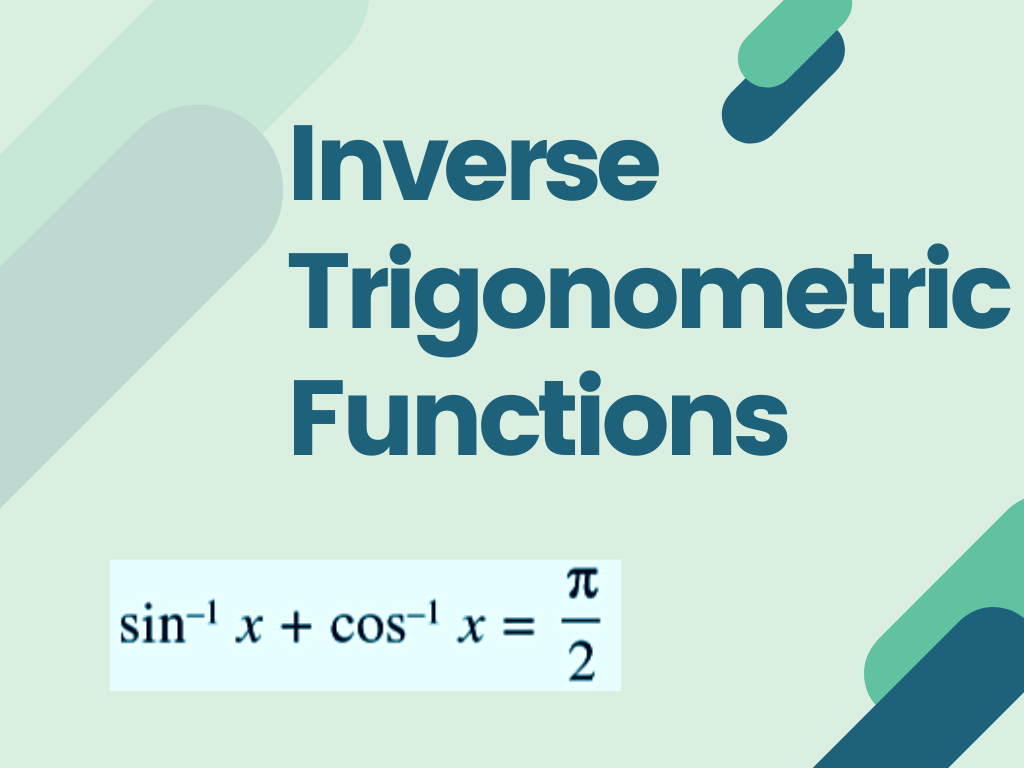# Relations and Functions## Inverse Trigonometric Functions Class 12th

Introduction Explanation Properties of Inverse Trigonometric Functions Related posts Trigonometry Class 10th Trigonometric Ratios of Acute Angles Class 10th Trigonometric Ratios of Some Specific Angles Class 10th Relation Between Trigonometric Ratios Class 10th Relations and Functions Class 12th कुछ विशिष्ट कोणों के लिए त्रिकोणमितीय अनुपात कक्षा 10 (Trigonometric Ratios of Specific Angles Class 10th) न्यून …## Relations and Functions Class 12th

Introduction The meaning of the word Relation in the English language is a connection or link between two objects. In mathematics, the same concept is used between two numbers or quantities. In the previous class, we studied Relations and Functions like cartesian products of sets, Domains, co-domain, ranges, Real functions, Identity functions, Constant functions, Polynomial functions, …